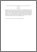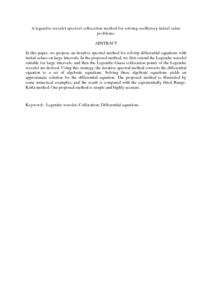# A legendre wavelet spectral collocation method for solving oscillatory initial value problems.

## Citation

Dizicheh, Ali Karimi and Ismail, Fudziah and Kajani, M. Tavassoli and Maleki, Mohammad (2013) A legendre wavelet spectral collocation method for solving oscillatory initial value problems. Journal of Applied Mathematics, 2013 (591636). pp. 1-5. ISSN 1110-757X; ESSN: 1687-0042

## Abstract

In this paper, we propose an iterative spectral method for solving differential equations with initial values on large intervals. In the proposed method, we first extend the Legendre wavelet suitable for large intervals, and then the Legendre-Guass collocation points of the Legendre wavelet are derived. Using this strategy, the iterative spectral method converts the differential equation to a set of algebraic equations. Solving these algebraic equations yields an approximate solution for the differential equation. The proposed method is illustrated by some numerical examples, and the result is compared with the exponentially fitted Runge-Kutta method. Our proposed method is simple and highly accurate.Preview
PDF (Abstract)
A legendre wavelet spectral collocation method for solving oscillatory initial value problems.pdfView Item